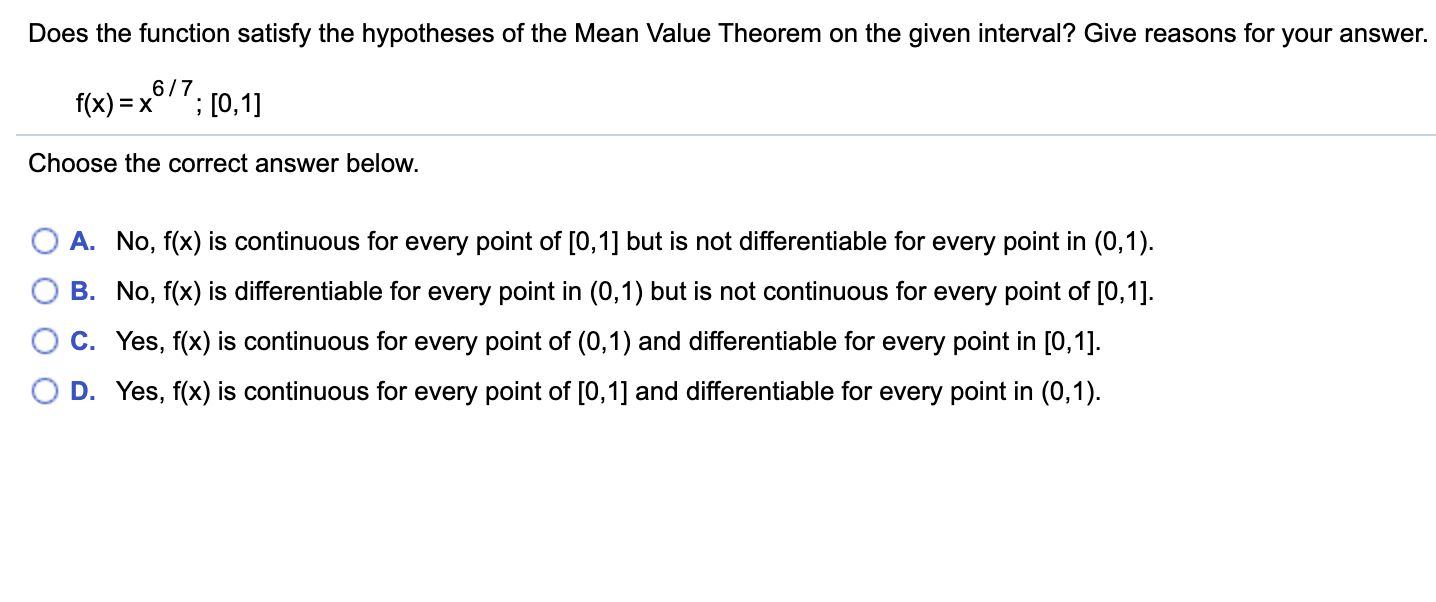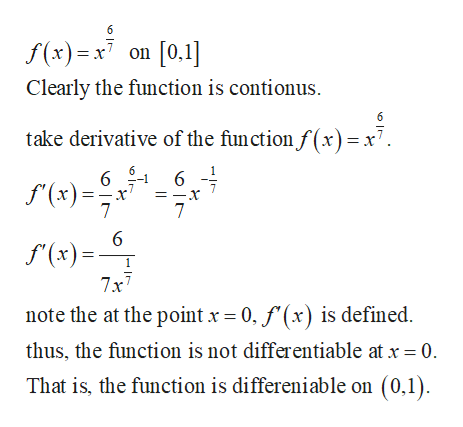# Does the function satisfy the hypotheses of the Mean Value Theorem on the given interval? Give reasons for your answer.6/7f(x) = x;[0,1]Choose the correct answer below.A. No, f(x) is continuous for every point of [0,1] but is not differentiable for every point in (0,1)B. No, f(x) is differentiable for every point in (0,1) but is not continuous for every point of [0,1]C. Yes, f(x) is continuous for every point of (0,1) and differentiable for every point in [0,1].D. Yes, f(x) is continuous for every point of [0,1] and differentiable for every point in (0,1)

Question
13 views

Can you help me step by step?help_outlineImage TranscriptioncloseDoes the function satisfy the hypotheses of the Mean Value Theorem on the given interval? Give reasons for your answer. 6/7 f(x) = x ;[0,1] Choose the correct answer below. A. No, f(x) is continuous for every point of [0,1] but is not differentiable for every point in (0,1) B. No, f(x) is differentiable for every point in (0,1) but is not continuous for every point of [0,1] C. Yes, f(x) is continuous for every point of (0,1) and differentiable for every point in [0,1]. D. Yes, f(x) is continuous for every point of [0,1] and differentiable for every point in (0,1) fullscreen
check_circle

Step 1

Check:

...help_outlineImage Transcriptionclose6 f(x)x7on [0.1] Clearly the function is contionus 6 take derivative of the function f (x)x7. 6 f(x) 7 7 6 f(x) 7x7 note the at the point x 0, f (x) is defined thus, the function is not differentiable at x = 0 That is, the function is differeniable on (0,1). fullscreen

### Want to see the full answer?

See Solution

#### Want to see this answer and more?

Solutions are written by subject experts who are available 24/7. Questions are typically answered within 1 hour.*

See Solution
*Response times may vary by subject and question.
Tagged in

### Calculus